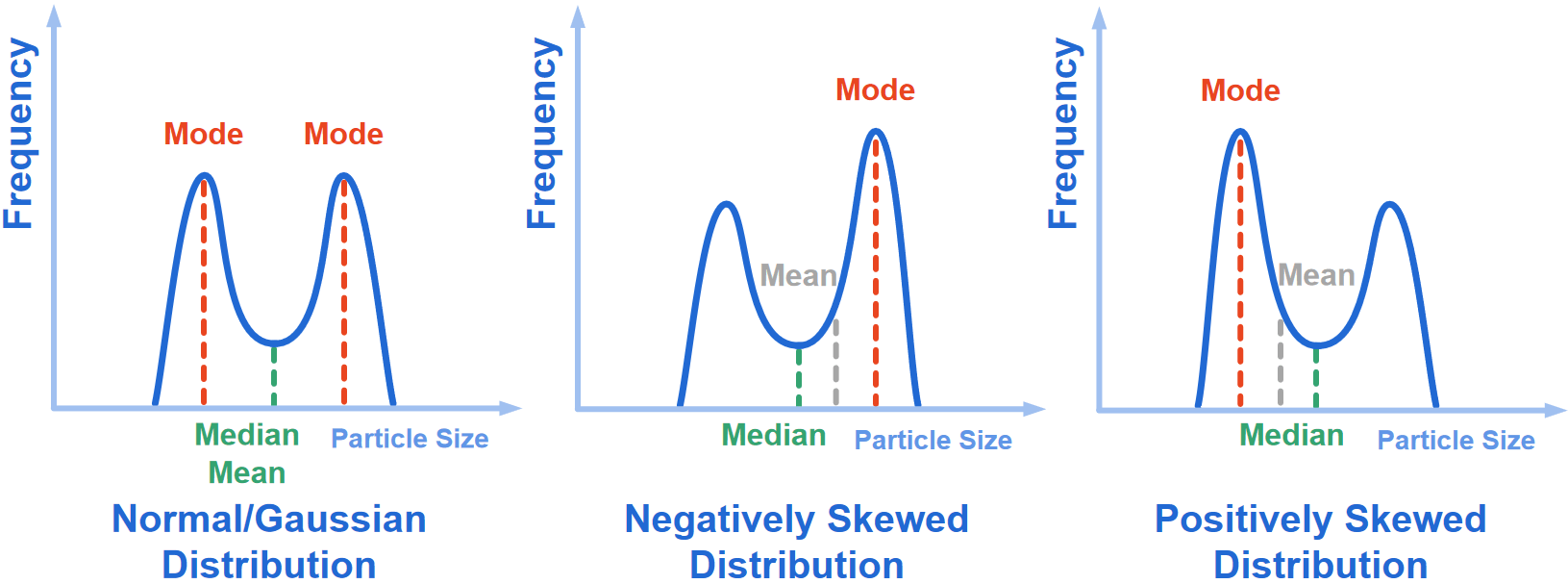Hmoe > Learn > Wiki > How to determine mean, median, and mode?

# How to determine mean, median, and mode?

2023-07-12WIKI

The median is the middle value in a list of values ordered from the smallest value to the largest value.

The mode is the value that most frequently occurs on the list of values.

The mean is calculated by adding the values on the list and dividing the total sum of values by the number of values on the list. This is best described by the formula below.

The mean, denoted  ¯x , of a set of n values x1, x2, ...xn,  is defined as the sum of the numbers divided by  :From the normal/Gaussian distribution shown below, it can be seen that the mean, median, and mode have all got the same value. Also, a normal/Gaussian distribution has values that the nearer they are to the mean, they will occur more frequently than the values that are further away from the mean. So, the mean value = median value = mode value = D50 value = 50% value.

The values for mode and mean are different to the median for negatively and positively skewed distributions.Normal/Gaussian distribution, negatively and positively skewed distributions for a bimodal distribution are shown below.Struggling to grasp particle analysis concepts? Our new Beginner's Guide to Particle Analysis is here to help you grasp these concepts in the most accessible manner possible. Download it as a PDF to read on the train, on the plane, or wherever else there’s no internet.